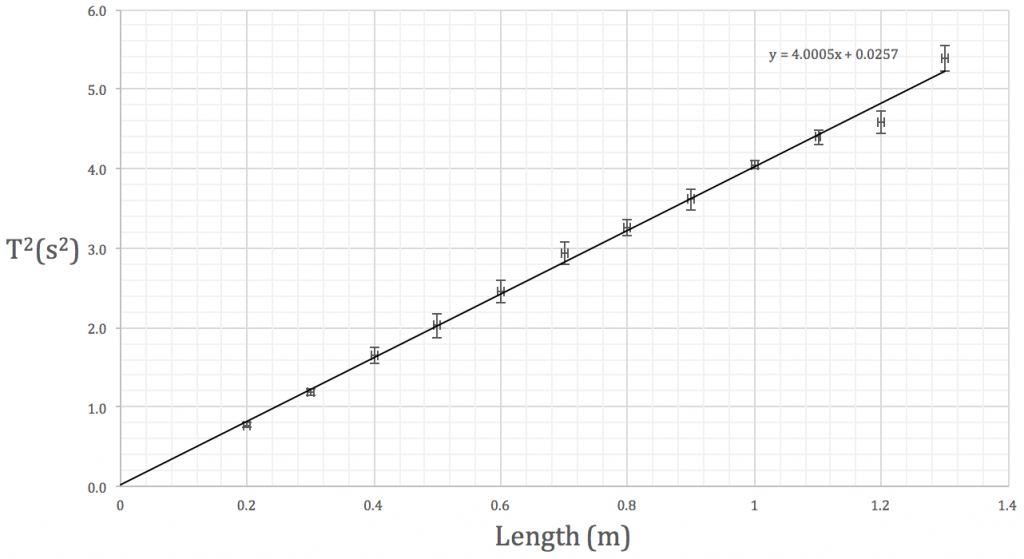## nat5 revision

Use the attached file to help with revision for your test on Monday.  Start at the beginning of the booklet and read up to the end of page 41.

Thanks to Mr Noble for sharing his notes. 🙂

## nat5 – work, power and potential energy

We’ve been looking at work and the rate of change of energy.  This handout will help you to revise the key points and introduces gravitational potential energy.

## using Excel’s LINEST function

The period T of a simple pendulum can be calculated using$T=2 \pi \displaystyle \sqrt{l \over g}$

where l is the pendulum length and g is the gravitational field strength.

Using a single value of length and period, we can determine the acceleration due to gravity.  However, it would be better experimental practise to vary the length of the pendulum and plot a graph of$T^2$ against length, determining g from the gradient of the line of best fit.We’re going to spend the next three periods analysing your simple pendulum data in the library.  The attached pdf will walk you through the steps.  It would be better if you used your own results but I’ve put some sample data on the first page if you’ve forgotten to bring yours.

## nat5 – waves and radiation summary notes

Here are whole unit summary notes to help you prepare for the unit test next week.

Thanks to Mr Noble for sharing his notes.